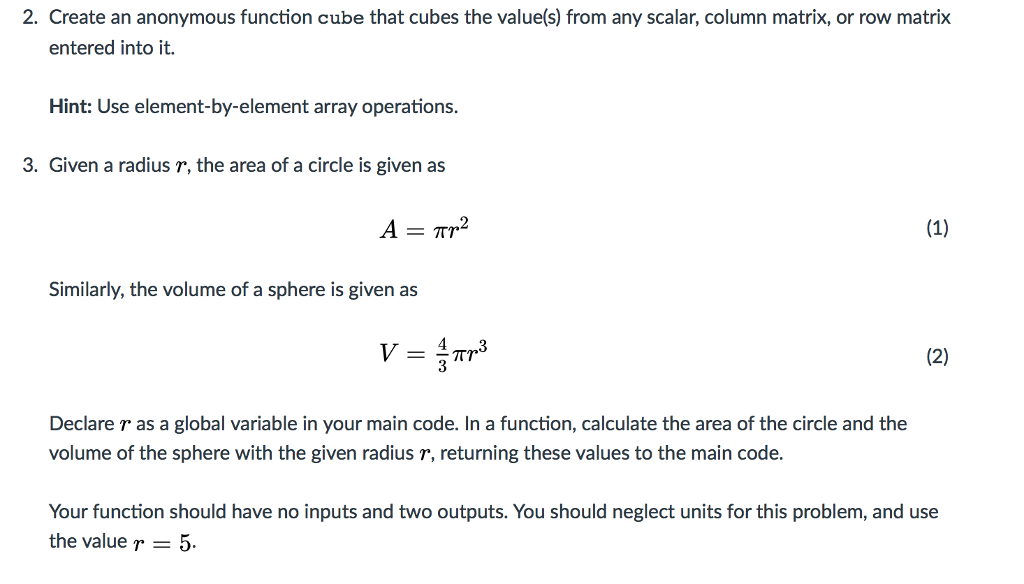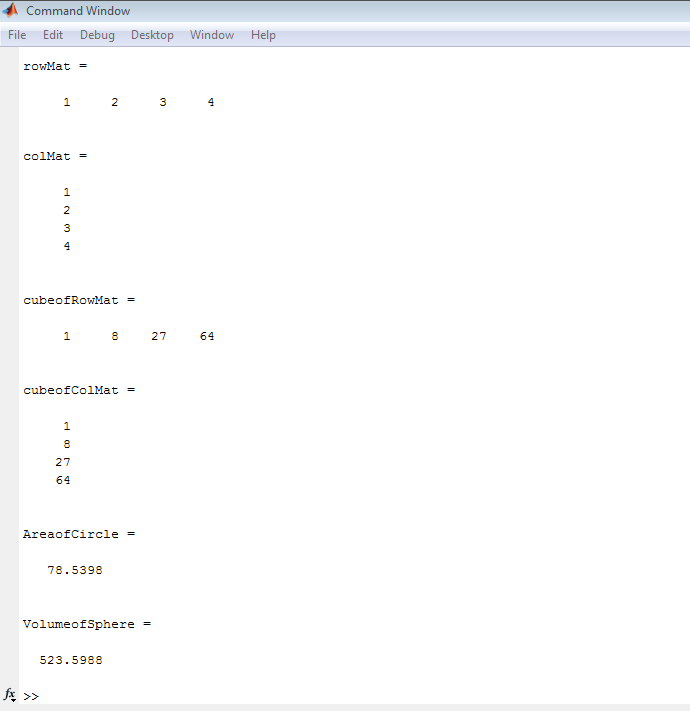# Answered! Create a single script (.m file) to complete this assignment, plus such separate function file(s) (.m file(s)) as are…

Create a single script (.m file) to complete this assignment, plus such separate function file(s) (.m file(s)) as are requested in the prompts. Unless directed otherwise, use meaningful variable names for each variable; do not use the default variable ans to store your results. For this assignment do not suppress your output with semi-colons (;). The main script code for each problem (i.e. Problem 2 and Problem 3) should be in a separate cell of your script, using the cell mode feature of MATLAB. Your submission must be a single script plus such separate function file(s) as are requested in the prompts.Don't use plagiarized sources. Get Your Custom Essay on
Answered! Create a single script (.m file) to complete this assignment, plus such separate function file(s) (.m file(s)) as are…
GET AN ESSAY WRITTEN FOR YOU FROM AS LOW AS \$13/PAGE

THE SCRIPT FILE BELOW

%% —————————————————————–

global r
r=5;
cube=@(x) x.^3;
%% row matrix
rowMat=[1,2,3,4]
% Column matrix
colMat=[1;2;3;4]
% Cube of row matrix
cubeofRowMat=cube(rowMat)
%Cube of row matrix
cubeofColMat=cube(colMat)
%% Call to compute area of circle
AreaofCircle=areaCircle()
%% Call to compute volume of shpere
VolumeofSphere=volumeSphere()

——————————————————————————————————-

Funtion file : areaCircle.m

——————————————————————–

function A = areaCircle()
%areaCircle return the area
% of a circle with given global radius r
global r;
A=pi*r*r;
end

———————————————————————————–

function File :volumeSphere.m

——————————————————-

function V =volumeSphere()
%volumeSphere return the volume
% of a sphere with given global radius r
global r;
V=pi*r*r*r*4/3;

end

—————————————————————————-

command window output

——————————————————————